In :
%pylab inline
from scipy import signal

Populating the interactive namespace from numpy and matplotlib


This notebook contains a detailed description of implementing classic second order filter sections using matrix arithmetic. The matrix formulation lends itself to robust, fast evaluation on SIMD architectures. Second order filter sections are extremely versatile, as a single section can implement many different types of filter, including lowpass, highpass, bandpass, notch, bell, low shelf, and high shelf. Being second order, rolloff is 12dB/octave. For sharper frequency response, higher order IIR filters can be decomposed into multiple second order sections.

All second order filters can be described using two state variables. The evolution of the state is a 2x2 matrix. In addition, there is a 2-vector describing the contribution of the input to the state, a 2-vector describing "reading out" the state to the output, and a scalar for the zero-delay contribution of the input to the output. For mathematical convenience, we group the last two into a single 3-vector. Thus, a filter is described as the tuple $(\mathbf{A}, B, C)$, and each step is as follows:

\begin{align} out_n & = C \cdot concat([[x_n], y_n]) \\ y_{n+1} & = B \cdot x_n + \mathbf{A} \cdot y_n \\ \end{align}

Where the $\cdot$ notation represents dot product, vector times scalar, or matrix times vector multiplication, respectively.

Question: would it be more convenient to have a single 3x3 matrix?

There is some redundancy in this representation. Obviously, multiplying B times some scalar, and dividing C by the same, is a filter with the same results. More subtly, the y vector can be transformed by any non-degenerate linear transformation. However, these transforms do matter when the filter parameters are modulated, which is especially common in synthesizers, and also matter for roundoff behavior (particularly important when evaluating the filters using 32 bit floating point arithmetic).

The reference code for evaluating a filter in this form is straightforward:

In :
def eval(filt, inp):
A, B, C = filt
result = []
y = zeros(2)
for x in inp:
result.append(dot(C, concatenate([[x], y])))
y = B * x + dot(A, y)
return array(result)


Now how do we get a filter in this form? Starting from standard biquad coefficients is, again, pretty easy. Here, the y vector represents the two state variables in transposed direct form II. For review, the transfer function of this filter is:

$$H(z) = \frac{b_0 + b_1 z^{-1} + b_2 z^{-2}}{1 + a_1 z^{-1} + a_2 z^{-2}}$$
In :
def from_biquad(coefs):
b0, b1, b2, a1, a2 = coefs
A = array([[-a1, 1], [-a2, 0]])
B = array([b1 - a1 * b0, b2 - a2 * b0])
C = array([b0, 1, 0])
return A, B, C


We can test this against a straightforward implementation (in this case, direct form I).

In :
def biquad(coefs, inp):
b0, b1, b2, a1, a2 = coefs
out = zeros(len(inp))
x1 = 0
x2 = 0
y1 = 0
y2 = 0
for i in range(len(inp)):
x = inp[i]
y = b0 * x + b1 * x1 + b2 * x2 - a1 * y1 - a2 * y2
out[i] = y
x2, x1 = x1, x
y2, y1 = y1, y
return out

impulse = zeros(100)
impulse = 1
coefs = 1.0207, -1.7719, .9376, -1.7719, 0.9583  # peaking
show()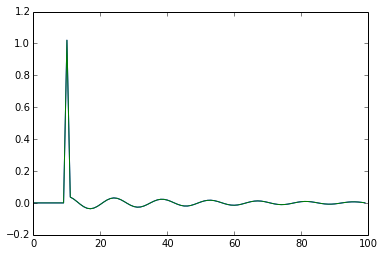## State Variable Filter design¶

However, biquads are not necessarily your best bet for designing second order filters, for two reasons. First, they don't modulate well, because the state space for one set of filter parameters probably doesn't make sense with a different filter. Second, roundoff behavior can be bad. We'll analyze that in more detail later.

A much better design paradigm is to discretize the State Variable Filter. Historically, many people used the naive discretization, originally popularized by Hal Chamberlin's book, Musical Applications of Microprocessors (see https://ccrma.stanford.edu/~jos/svf/svf.pdf), but this only works well at low frequencies and becomes unstable for frequencies around one sixth the sampling rate, or even worse for higher resonance values. Fortunately, Andrew Simper of Cytomic has done a thorough development of discretization using trapezoidal integration. This is equivalent to the bilinear transform, which is not exactly trivial because the trapezoidal integration (unlike the naive discretization) contains a delay-free loop. But fortunately Simper has done all the work for us:

Solving the continuous SVF equations using trapezoidal integration and equivalent currents

For simplicity, we'll start with just a lowpass filter. As we'll see later, the full derivation is very general and can do the entire RBJ suite of filter designs.

In :
def svf_lp(f, res):
g = tan(pi * f)
k = 2 - 2 * res # 1/Q
a1 = 1/(1 + g * (g + k))
a2 = g * a1
a3 = g * a2
A = array([[2*a1 - 1, -2*a2],
[2*a2, 1 - 2*a3]])
B = array([2 * a2, 2 * a3])
C = array([a3, a2, 1 - a3])
return A, B, C


Simper prefers a resonance control that ranges from 0 to 1. If you prefer the classic Q, just use this conversion:

$$res = 1 - .5/Q$$

Let's test the filter out:

In :
def db(x):
return 20 * log(x)/log(10)
def plot_fr(filter):
impulse = concatenate([, zeros(5000)])
w, h = signal.freqz(eval(filter, impulse))
semilogx(w, db(abs(h)))
axis([3e-3, pi, -60, 10])
for f in [0.00316, 0.01, 0.0316, 0.1, 0.316, 0.49]:
plot_fr(svf_lp(f, 0.293))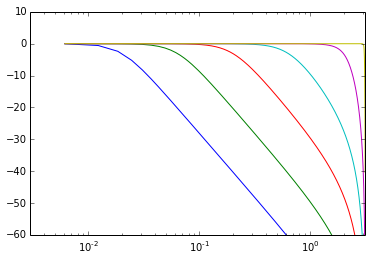This is the classic BLT lowpass filter response, with complete attenuation at Nyquist and the characteristic frequency warping.

In :
axis([3e-3, pi, -60, 10])
for f in [0.00316, 0.01, 0.0316, 0.1, 0.316, 0.49]:
plot_fr(svf_lp(f, 0.8))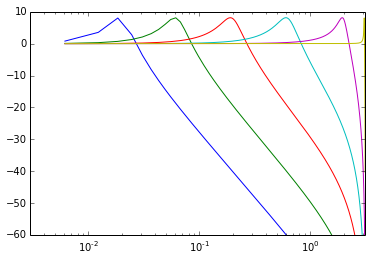As promised, the rest of the RBJ designs.

In :
def svf_core(f, res, m, shelfA = 1):
k = 2 - 2 * res
g = tan(pi * f) * shelfA
a1 = 1/(1 + g * (g + k))
a2 = g * a1
a3 = g * a2
A = array([[2*a1 - 1, -2*a2],
[2*a2, 1 - 2*a3]])
B = array([2 * a2, 2 * a3])
C_v0 = array([1, 0, 0])
C_v1 = array([a2, a1, -a2])
C_v2 = array([a3, a2, 1 - a3])
C = m * C_v0 + m * C_v1 + m * C_v2
return A, B, C

def svf_lp(f, res):
return svf_core(f, res, [0, 0, 1])

def svf_bp(f, res):
return svf_core(f, res, [0, 1, 0])

def svf_hp(f, res):
k = 2 - 2 * res
return svf_core(f, res, [1, -k, -1])

def svf_notch(f, res):
k = 2 - 2 * res
return svf_core(f, res, [1, -k, 0])

def svf_peak(f, res):
k = 2 - 2 * res
return svf_core(f, res, [1, -k, -2])

def svf_bell(f, Q, gaindB):
A = 10 ** (gaindB / 40.)
k = 1/(Q * A)
return svf_core(f, 1 - 0.5 * k, [1, k * (A * A - 1), 0])

def svf_lowshelf(f, Q, gaindB):
A = 10 ** (gaindB / 40.)
k = 1./Q
return svf_core(f, 1 - 0.5 * k, [1, k * (A - 1), A * A - 1], 1/sqrt(A))

def svf_highshelf(f, Q, gaindB):
A = 10 ** (gaindB / 40.)
A2 = A * A
k = 1./Q
return svf_core(f, 1 - 0.5 * k, [A2, k * (A - A2), 1 - A2], sqrt(A))

In :
axis([3e-3, pi, -60, 10])
f0 = 0.013
res = 0.293
plot_fr(svf_lp(f0, res))
plot_fr(svf_bp(f0, res))
plot_fr(svf_hp(f0, res))
plot_fr(svf_notch(f0, res))
plot_fr(svf_peak(f0, res))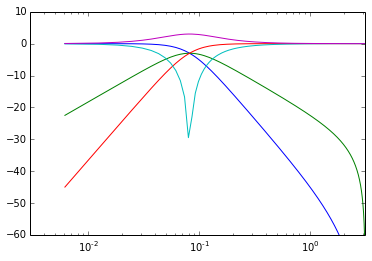In :
axis([3e-3, pi, -40, 40])
for gain in range(-30, 31, 10):
plot_fr(svf_bell(f0, .5, gain))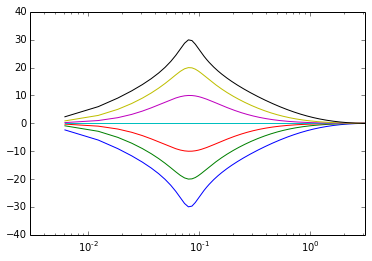In :
axis([3e-3, pi, -40, 40])
for gain in range(-30, 31, 10):
plot_fr(svf_lowshelf(f0, .707, gain))
plot_fr(svf_highshelf(f0, .707, gain))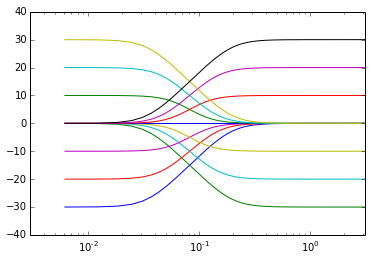## Fast matrix evaluation¶

The matrix form above is reasonably efficient to implement. However, to maximally take advantage of the power of modern SIMD, we can construct a single 4x4 matrix that computes two samples in a single iteration. Thus, the iteration of the state is represented by the square of the $\mathbf{A}$ matrix above.

In :
def mk4mat(filter):
A, B, C = filter
AA = dot(A, A)
AB = dot(A, B)
CA = dot(C[1:], A)
cb = dot(C[1:], B)
return array([[C, 0, C, C],
[cb, C, CA, CA],
[AB, B, AA, AA],
[AB, B, AA, AA]])


Then, evaluation is again quite simple, each iteration processing two samples. The central 4x4 matrix-vector multiply is especially well suited for implementation in NEON, see cpp/src/neon_iir.s for NEON assembly language.

In :
def eval4(A, inp):
out = zeros(len(inp))
y = zeros(4)
for i in range(0, len(inp), 2):
y[0:2] = inp[i:i+2]
y = dot(A, y)
out[i:i+2] = y[0:2]
return out


Let's test it works the same:

In :
filter = svf_bp(f0, res)
plot(eval(filter, impulse))
plot(eval4(mk4mat(filter), impulse))
show()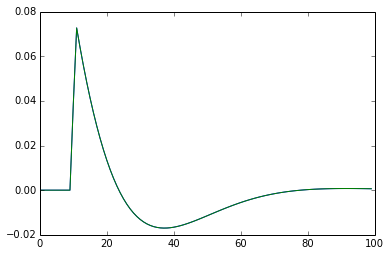With the 4x4 matrix formulation, each iteration performs 16 multiplies and 12 adds to compute two samples, or 8 multiplies and 6 adds per sample. This is slightly better than the 9 multiplies and 8 adds for the most general form of Simper's code, and roughly the same as most of the specialized forms. However, raw number of multiply/add operations tells only a small part of the speed story. All these operations are grouped for easy computation in 4x wide SIMD instructions. On NEON, this yields 1.47ns per IIR on a Nexus 5, which is 6x a straightforward scalar implementation of direct form I biquad evaluation.

## Error measurement¶

We want to evaluate these filters in floating point arithmetic for speed. IIR filters are notoriously finicky about roundoff error.

For these tests, we'll run a filter in double math, then the same filter in float, and compare.

In :
def eval4_f32(A, inp):
A = array(A, dtype=float32)
inp = array(inp, dtype=float32)
out = zeros(len(inp), dtype=float32)
y = zeros(4, dtype=float32)
for i in range(0, len(inp), 2):
y[0:2] = inp[i:i+2]
y = dot(A, y)
out[i:i+2] = y[0:2]
return out

In :
impulse = concatenate([, zeros(99)])
filter = mk4mat(svf_lp(.1, .75))
plot(eval4(filter, impulse) - eval4_f32(filter, impulse))
grid(True)
show()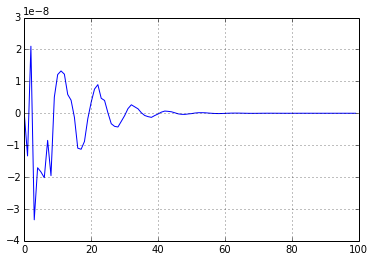For comparison, here's a similar test with biquad:

In :
# Adapted directly from Audio EQ Cookbook, by Robert Bristow-Johnson
def rbj_lpf(f, res):
w0 = 2 * pi * f
Q = 1/(2 - 2 * res)
cw0 = cos(w0)
b1 = 1 - cw0
b0 = .5 * b1
b2 = b0
alpha = sin(w0)/(2*Q)
a0 = 1 + alpha
a1 = -2 * cw0
a2 = 1 - alpha
ra0 = 1/a0
return b0 * ra0, b1 * ra0, b2 * ra0, a1 * ra0, a2 * ra0

b0, b1, b2, a1, a2 = map(float32, coefs)
out = zeros(len(inp), dtype = float32)
x1 = float32(0)
x2 = float32(0)
y1 = float32(0)
y2 = float32(0)
for i in range(len(inp)):
x = float32(inp[i])
y = b0 * x + b1 * x1 + b2 * x2 - a1 * y1 - a2 * y2
out[i] = y
x2, x1 = x1, x
y2, y1 = y1, y
return out


We'll design the same filter in both biquad and SVF forms, then check that the impulse response is the same.

In :
coefs = rbj_lpf(.1, .75)
filter = mk4mat(svf_lp(.1, .75))
plot(eval4(filter, impulse))
grid(True)
show()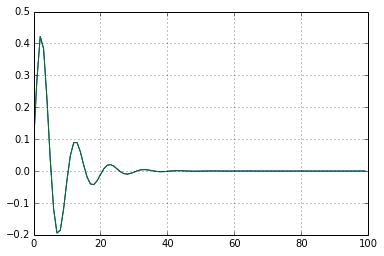And we can see that the error is much less with the matrix state variable approach.

In :
plot(biquad(coefs, impulse) - biquad_f32(coefs, impulse))
plot(eval4(filter, impulse) - eval4_f32(filter, impulse))
grid(True)
show()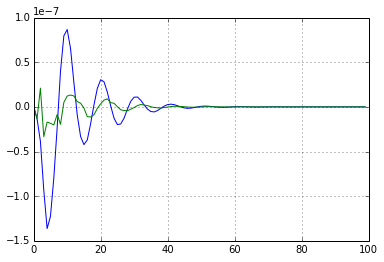The difference is even more dramatic at lower frequencies, where the error of direct form biquad evaluation is large.

In :
impulse = concatenate([, zeros(499)])
coefs = rbj_lpf(0.01, .75)
filter = mk4mat(svf_lp(0.01, .75))
plot((eval4(filter, impulse) - eval4_f32(filter, impulse)))
grid(True)
show()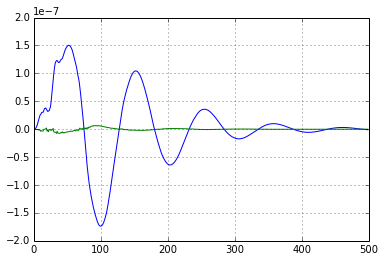By visualizing the state of the filter, it's possible to see intuitively where the difference in numerical robustness comes from. In the SVF, where the state corresponds to physical quantities, the norm of the state vector is close to the system's energy.

In :
def eval_showstate(filt, inp):
A, B, C = filt
result = []
y = zeros(2)
for x in inp:
result.append(y)
y = B * x + dot(A, y)
return result

impulse = concatenate([, zeros(49)])
filt = svf_lp(0.02, 0.5)
xs, ys = zip(*eval_showstate(filt, impulse))
axes().set_aspect(1)
grid(True)
plot(xs, ys, '-o')

Out:
[<matplotlib.lines.Line2D at 0x1094aa250>]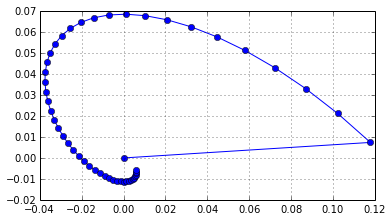By contrast, with biquad, the state vector is a fairly extreme transform. Thus, even a relatively small error in the state variables can lead to a significant delta in energy, resulting in much larger output errors. The transform becomes more extreme as the frequency decreases. Note that this is the biquad in matrix form, which is most similar to transposed direct form II. However, error behavior is broadly similar, at least in floating point, for all the biquad evaluation schemes.

In :
filt = from_biquad(rbj_lpf(0.02, 0.5))
xs, ys = zip(*eval_showstate(filt, impulse))
axes().set_aspect(1)
grid(True)
plot(xs, ys, '-o')

Out:
[<matplotlib.lines.Line2D at 0x10974ccd0>]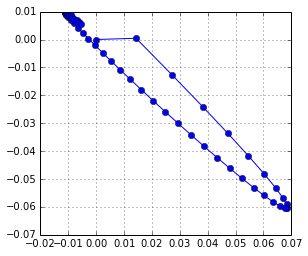## Modulation¶

The main reason to prefer one state space over another for the same transfer function is performance under modulation, that is when the filter parameters don't stay constant. Biquad filters are notoriously bad at this. State variable filters perform well under modulation and are highly regarded for these use cases.

Let's test it and see how it does.

In :
def saw(freq, n): return 1-2 * (linspace(0, freq * n, num=n, endpoint=False) % 1)
def sweep(n, maxf = 0.5):
out = zeros(n)
ph = 0
for i in range(n):
out[i] = sin(ph)
f = exp(5 * (float(i) / n - 1)) * pi * 2 * maxf
ph += f
return out

In :
def eval_mod(freq, res, inp, filtf):
A, B, C = filt
result = []
y = zeros(2)
for i in range(len(inp)):
x = inp[i]
A, B, C = filtf(freq[i], res)
result.append(dot(C, concatenate([[x], y])))
y = B * x + dot(A, y)
return result


The spectrogram looks good.

In :
n = 10000
res = 0.9
specgram(eval_mod(0.25 + 0.2 * sweep(n, 0.1), res, saw(0.05, n), svf_lp))
show()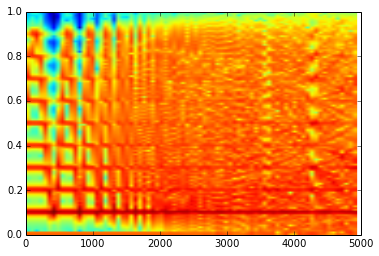By contrast, the spectrum of the modulated biquad is a lot dirtier and has high energy artifacts at high modulation rates. The problem gets worse at higher resonance settings.

In :
def rbj_lpf_mat(f, res):
specgram(eval_mod(0.25 + 0.2 * sweep(n, 0.1), res, saw(0.05, n), rbj_lpf_mat))
show()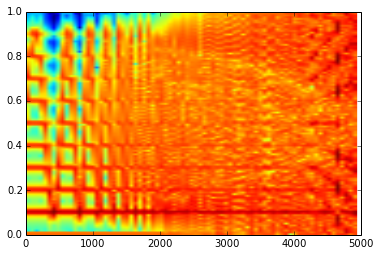It's visually even easier to see when the modulation has sharp steps in it.

In :
res = 0.1
specgram(eval_mod(0.15 + 0.1 * sign(sweep(n, 0.1)), res, saw(0.05, n), svf_lp))
show()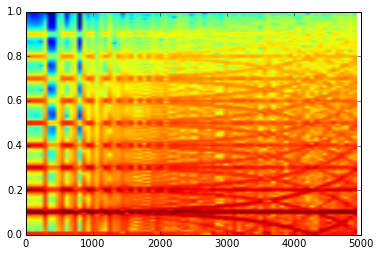In :
specgram(eval_mod(0.15 + 0.1 * sign(sweep(n, 0.1)), res, saw(0.05, n), rbj_lpf_mat))
show()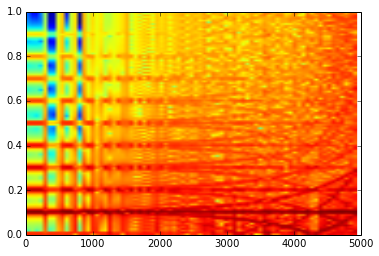With very high modulation, the biquad can actually become unstable, while the state variable formulation performs fine.

In :
r = 0.9
specgram(eval_mod(0.25 + 0.185 * sign(sweep(n, 0.1)), res, saw(0.05, n), svf_lp))
show()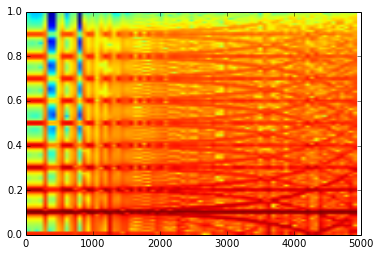In :
specgram(eval_mod(0.25 + 0.185 * sign(sweep(n, 0.1)), res, saw(0.05, n), rbj_lpf_mat))
show()